## Selina Concise Mathematics Class 10 ICSE Solutions Chapter 7 Ratio and Proportion (Including Properties and Uses) Ex 7C

These Solutions are part of Selina Concise Mathematics Class 10 ICSE Solutions. Here we have given Selina Concise Mathematics Class 10 ICSE Solutions Chapter 7 Ratio and Proportion Ex 7C.

Other Exercises

Question 1.
If a : b = c : d, prove that:
(i) 5a + 7b : 5a – 7b = 5c + 7d : 5c – 7d.
(ii) (9a + 13b) (9c – 13d) = (9c + 13d) (9a – 13b).
(iii) xa + yb : xc + yd = b : d.
Solution: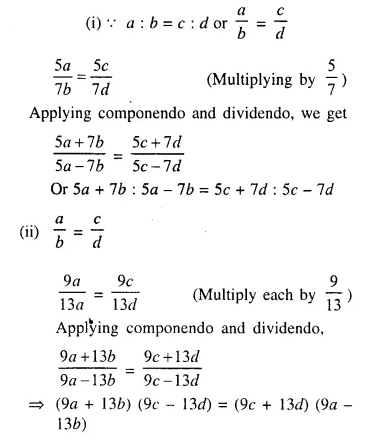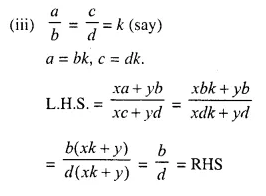Question 2.
If a : b = c : d, prove that: (6a + 7b) (3c – 4d) = (6c + 7d) (3a – 4b).
Solution: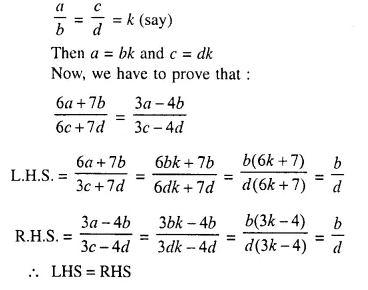Question 3.Solution: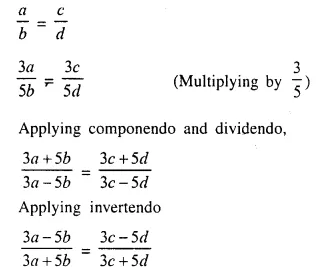Question 4.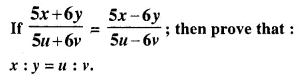Solution: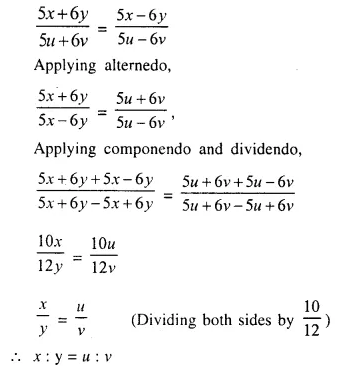Question 5.
If (7a + 8b) (7c – 8d) = (7a – 8b) (7c + 8d), prove that a : b = c : d.
Solution: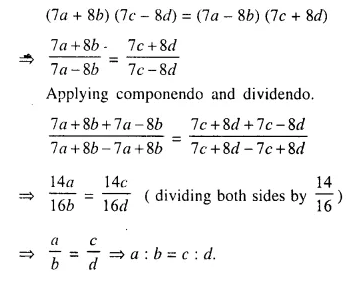Question 6.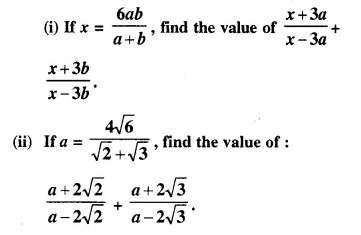Solution: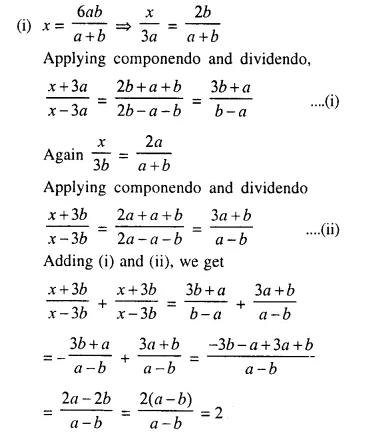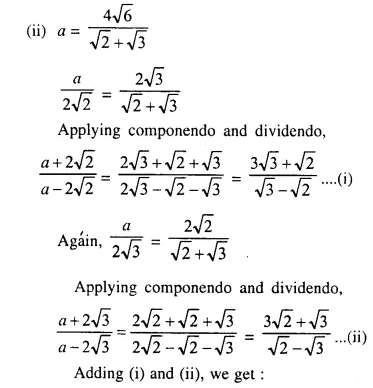Question 7.
If (a + b + c + d) (a – b – c + d) = (a + b – c – d) (a – b + c – d), prove that a : b = c : d.
Solution: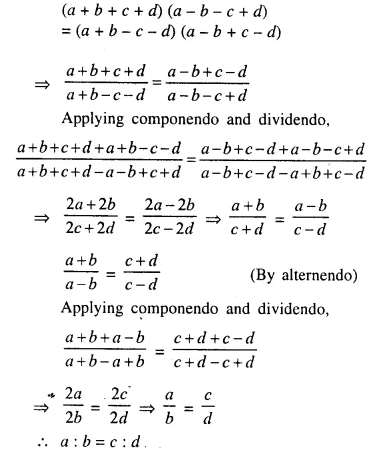Question 8.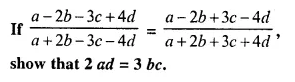Solution: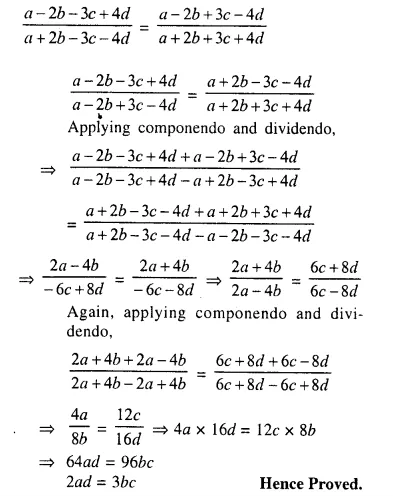Question 9.Solution: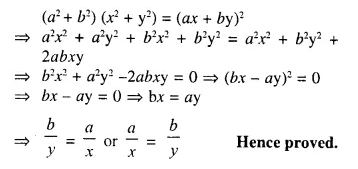Question 10.
If a, b and c are in continued proportion, prove that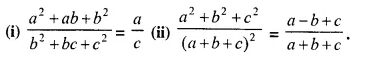Solution: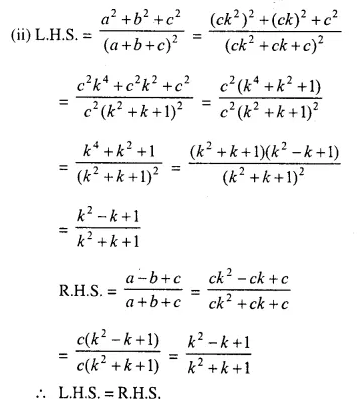Question 11.
Using properties of proportion, solve for x: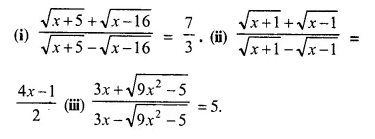Solution:But x = – 1 does not satisfy it
x = 1

Question 12.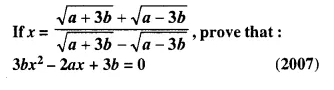Solution: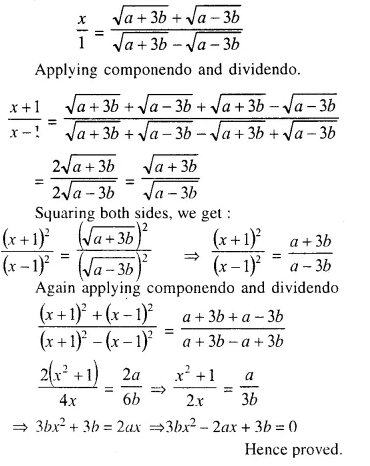Question 13.
Using the properties of proportion, solve for x, given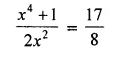Solution: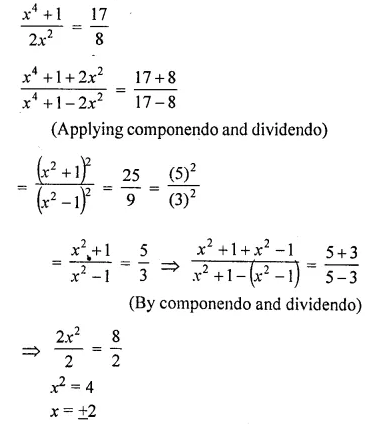Question 14.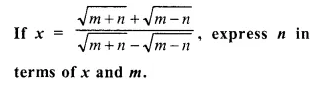Solution: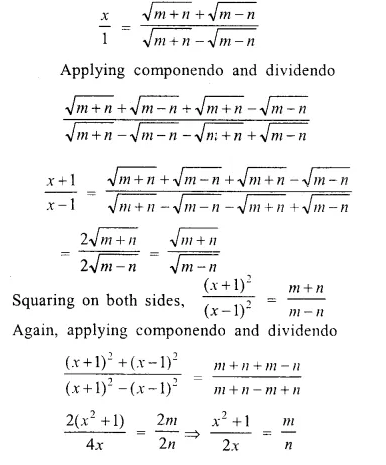Question 15.Solution: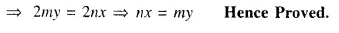Hope given Selina Concise Mathematics Class 10 ICSE Solutions Chapter 7 Ratio and Proportion Ex 7C are helpful to complete your math homework.

If you have any doubts, please comment below. Learn Insta try to provide online math tutoring for you.